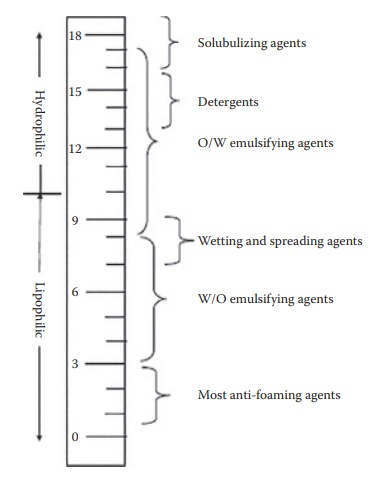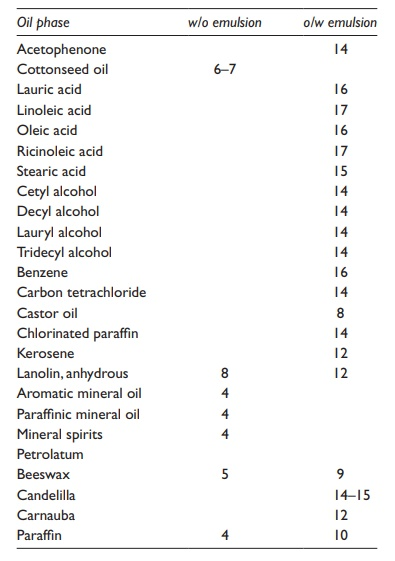# Hydrophile-lipophile balance system

| Home | | Pharmaceutical Drugs and Dosage | | Pharmaceutical Industrial Management |

## Chapter: Pharmaceutical Drugs and Dosage: Surfactants and micelles

Surfactants with the proper balance of hydrophilic and lipophilic affinities are effective emulsifying agents, since they concentrate at the oil–water inter-face, while being present in the two phases (oil and water) in different concentrations.

Hydrophile–lipophile balance system

In 1949, Griffin devised an arbitrary scale of values to serve as a measure of relative contributions of the hydrophilic and lipophilic regions of a surfac-tant to its overall hydrophilic/lipophilic character, which could be used to select emulsifying agents for a given application. This system is now widely known as the hydrophilelipophile balance (HLB) system. The higher the HLB value of an emulsifying agent, the more hydrophilic it is. The emul-sifying agents with lower HLB values are less polar and more lipophilic.

The HLB values of some commonly used surfactants are listed in Table 10.2. The Spans, that is, sorbitan esters, are lipophilic and have low HLB val-ues (1.8–8.6); the Tweens, poly(oxyethylene) derivatives of the Spans, are hydrophilic and have high HLB values (9.6–16.7). Figure 10.2 illustrates a scale showing surfactant function on the basis of HLB values. Utilizing this numbering system, it is possible to establish an HLB range of optimum efficiency for each application of surfactants.

Table 10.2 HLB values of commonly used surfactantsFigure 10.2 A scale showing surfactant function on the basis of HLB values.### 1. Type of emulsion formed

Surfactants with the proper balance of hydrophilic and lipophilic affinities are effective emulsifying agents, since they concentrate at the oil–water inter-face, while being present in the two phases (oil and water) in different con-centrations. Thus, a lipophilic surfactant would have higher concentration in oil, while a hydrophilic surfactant would have higher concentration in water. The phase with higher surfactant concentration tends to become the external phase in an emulsion. Thus, the HLB of a surfactant, or a combi-nation of surfactants, determines whether an o/w or w/o emulsion results. An emulsifying agent with high HLB is preferentially soluble in water and results in the formation of an o/w emulsion. The reverse situation is true with surfactants of low HLB value, which tend to form w/o emulsions. In general, o/w emulsions are formed when the HLB of the emulsifier is ~9–12, and w/o emulsions are formed when the HLB is ~3–6.

### 2. required HLB of a lipid

The lipid phase used in an emulsion can be assigned a required HLB (or RHLB) value. The RHLB for the lipid phase of an emulsion is the HLB value of the surfactant that provides the lowest interfacial tension between the two phases to form an o/w or a w/o emulsion. The RHLB, thus, provides guidance to surfactant selection for a specified lipid phase for the formation of a stable emulsion. The RHLB may be experimen-tally determined by preparing a series of emulsions with surfactants of different HLB values and selecting the HLB value that resulted in the physically most stable emulsion, as assessed, for example, by the separa-tion of phases on undisturbed storage of the emulsion. A list of RHLB values for common emulsifying agents is usually available in the litera-ture (Table 10.3).

Table 10.3 Required HLB for some oil-phase ingredients for making o/w and w/o emulsionsThis value is utilized in the HLB concept to prepare an emulsion by selecting an emulsifier that has the same, or nearly the same, HLB value as the RHLB of the oleaginous phase of the intended emulsion. For example, mineral oil has an assigned RHLB value of 4 if a w/o emulsion is desired, and it has a value of 10.5 if an o/w emulsion is desired.

### 3. required HLB of a formulation

The HLB values are additive. Therefore, calculation of the required HLB of a formulation is done by weighting the RHLB of each oil-phase ingredient (excluding any emulsifiers) as a weight percentage of the total oil-phase ingredients. For example, if the oil-phase ingredients of an o/w emulsion consist of 10% mineral oil, 3% capric/caprylic triglyceride, 2.5% isopropyl myristate, 4% cetyl alcohol, and the remaining emulsifiers, water, preser-vative, sweeteners, flavors, and colorants, the percentage oil-phase ingredi-ents in the formulation would be calculated as 10 + 3 + 2.5 + 4 = 19.5%. The RHLB of the oil phase for a desired o/w emulsion would be calculated as follows:Thus, the RHLB of the oil phase is 5.4 + 0.8 + 1.5 + 3.2 = 10.9. Hence, this formulation would require the use of an emulsifier, or a combination of emulsifiers, which should have the HLB of 10.9 to make an optimum physically stable o/w emulsion.

### 4. Assigning a hydrophile–lipophile balance value to a surfactant

The HLB value of a surfactant reflects a one-fifth fraction of the hydro-philic portion of the surfactant on a molecular-weight basis. For example, for calculating the HLB value of 22 moles of ethoxylate of oleyl alcohol, the molecular weight of 22 moles of ethylene oxide [−CH2−O−CH2−], with the repeating unit molecular weight of 44, is calculated to represent the hydro-philic portion of a surfactant. Thus, 22 × 44 = 968. This mass is added to the molecular weight of oleyl alcohol, 270, to get the total molecular weight of the surfactant. Thus, 968 + 270 = 1238. The percentage molecular weight of the surfactant that is hydrophilic is, therefore, 968/1238 × 100 = 77%.

Taking a one-fifth fraction, the HLB of this surfactant would be 77/5 = 15.4.

The HLB values are assigned only to nonionic surfactants. Thus, the HLB values are generally in the range of 0.5–19.5. Nevertheless, the HLB values of ionic surfactants are provided in the literature as an indication of their relative hydrophilicity. Some HLB values are listed in Table 10.2. Thus, an ionic surfactant with an HLB value of 40 simply indicates that it is highly hydrophilic.

### 5. Selection of surfactant combination for a target hydrophile–lipophile balance value

Frequently, a combination of two or more surfactants, usually with different molecular weight/size, is used instead of just one surfactant. A combination of surfactants ensures better packing at the interface and greater physical stability of the emulsion. In using a combination of surfactants, their HLB values are additive. Thus, the HLB value of a combination of surfactants is the weighted average of the HLB of each surfactant. For example, if 50% each of Span 20 and Span 80 were mixed together, the HLB of their com-bination would be 50/100 × 8.6 + 50/100 × 4.3 = 6.45. Similarly, the use of 90% Span 80 and 10 % Span 20 would give combined HLB value of 4.7, which is the same HLB value as that of Span 60. However, the use of Span 20 + Span 80 is expected to give a more stable emulsion than Span 60 in the same quantities.